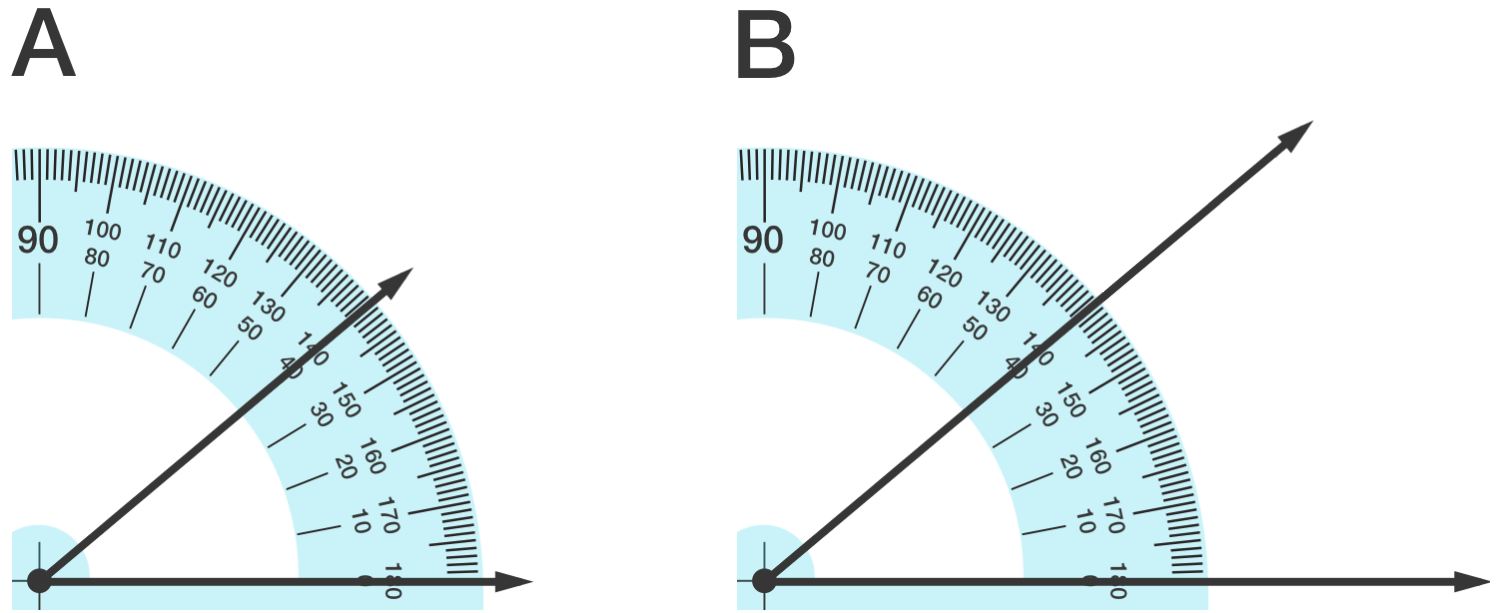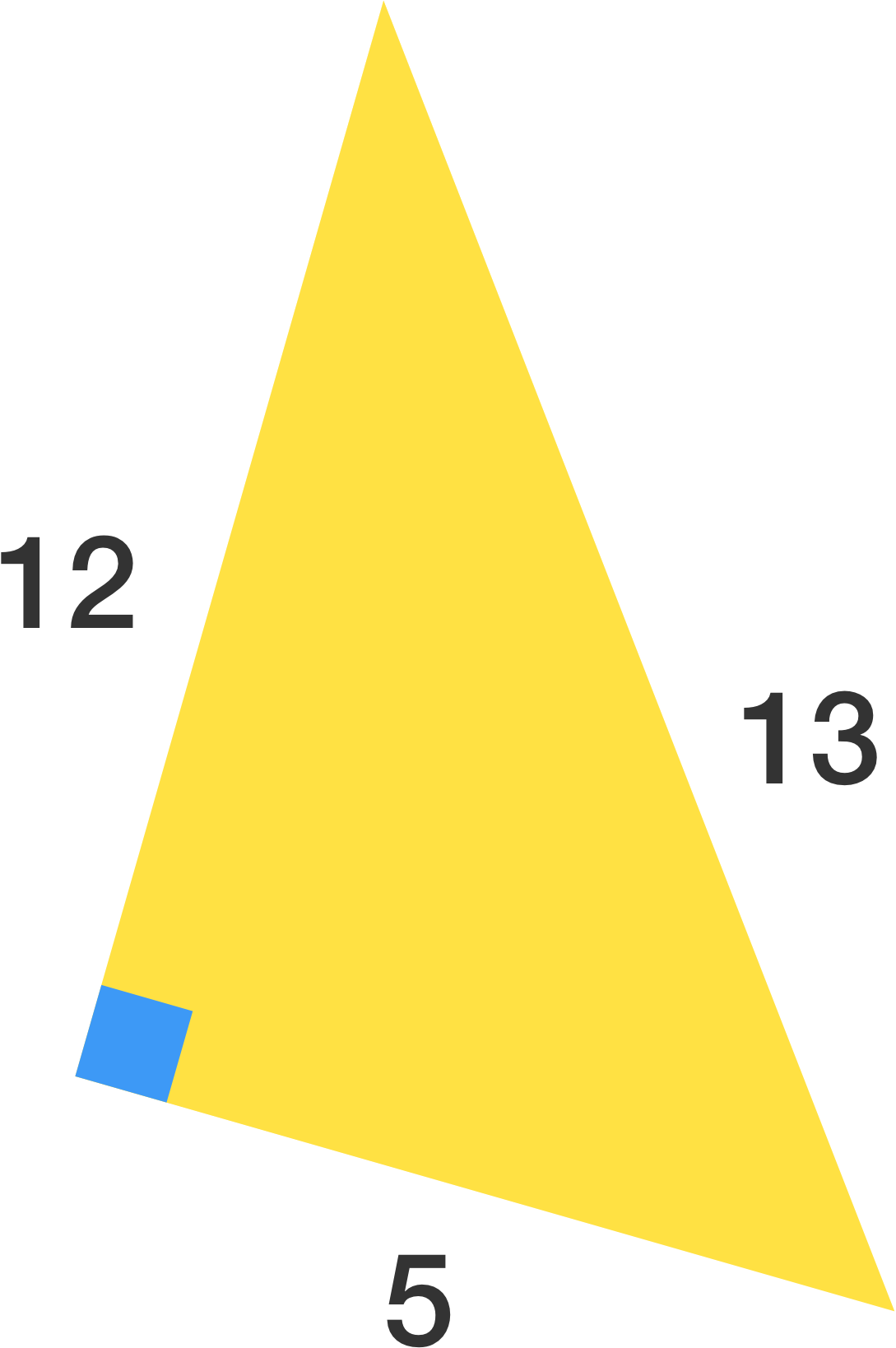Geometry

# Geometric Misconceptions WarmupRectangular pieces of square A are removed to obtain shape B. (They both still have the same overall height and width.) Which shape has the greater perimeter? (Note: All angles are right angles.)Which angle measure is bigger?

When using the standard formula to find the area of this triangle, $A = \frac{1}{2} bh$, which two side lengths should be used for the base and the height?Which statement is the converse of this statement: "If a quadrilateral is a square, then it is a rectangle"?

NOTE: While it might be, the converse of a statement is not necessarily true!

True or False?

A line can have a midpoint.

×